# 2D Translation in Computer Graphics | Definition | Examples

## 2D Transformation in Computer Graphics-

 In Computer graphics, Transformation is a process of modifying and re-positioning the existing graphics.

• 2D Transformations take place in a two dimensional plane.
• Transformations are helpful in changing the position, size, orientation, shape etc of the object.

### Transformation Techniques-

In computer graphics, various transformation techniques are-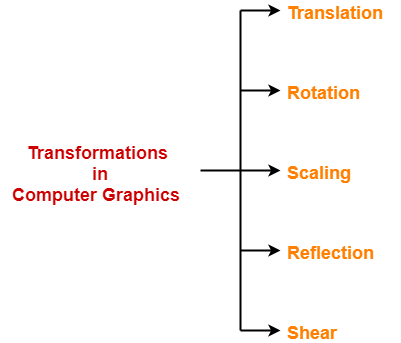In this article, we will discuss about 2D Translation in Computer Graphics.

## 2D Translation in Computer Graphics-

 In Computer graphics, 2D Translation is a process of moving an object from one position to another in a two dimensional plane.

Consider a point object O has to be moved from one position to another in a 2D plane.

Let-

• Initial coordinates of the object O = (Xold, Yold)
• New coordinates of the object O after translation = (Xnew, Ynew)
• Translation vector or Shift vector = (Tx, Ty)

Given a Translation vector (Tx, Ty)-

• Tx defines the distance the Xold coordinate has to be moved.
• Ty defines the distance the Yold coordinate has to be moved.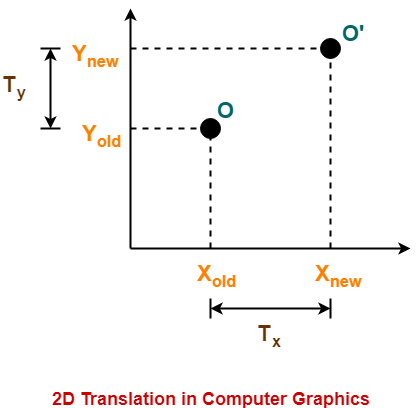This translation is achieved by adding the translation coordinates to the old coordinates of the object as-

• Xnew = Xold + Tx (This denotes translation towards X axis)
• Ynew = Yold + Ty (This denotes translation towards Y axis)

In Matrix form, the above translation equations may be represented as-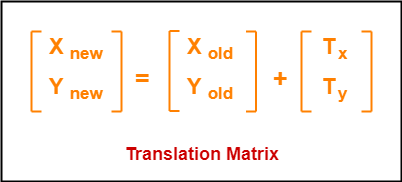• The homogeneous coordinates representation of (X, Y) is (X, Y, 1).
• Through this representation, all the transformations can be performed using matrix / vector multiplications.

The above translation matrix may be represented as a 3 x 3 matrix as-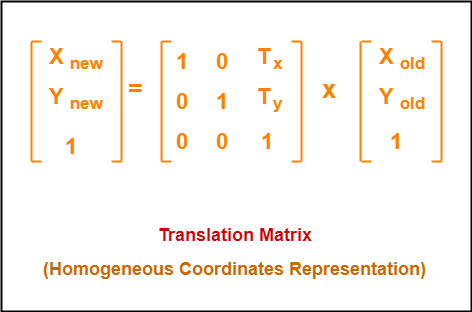## Problem-01:

Given a circle C with radius 10 and center coordinates (1, 4). Apply the translation with distance 5 towards X axis and 1 towards Y axis. Obtain the new coordinates of C without changing its radius.

## Solution-

Given-

• Old center coordinates of C = (Xold, Yold) = (1, 4)
• Translation vector = (Tx, Ty) = (5, 1)

Let the new center coordinates of C = (Xnew, Ynew).

Applying the translation equations, we have-

• Xnew = Xold + Tx = 1 + 5 = 6
• Ynew = Yold + Ty = 4 + 1 = 5

Thus, New center coordinates of C = (6, 5).

Alternatively,

In matrix form, the new center coordinates of C after translation may be obtained as-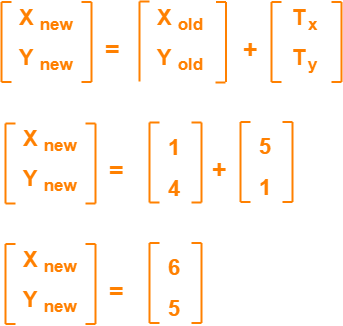Thus, New center coordinates of C = (6, 5).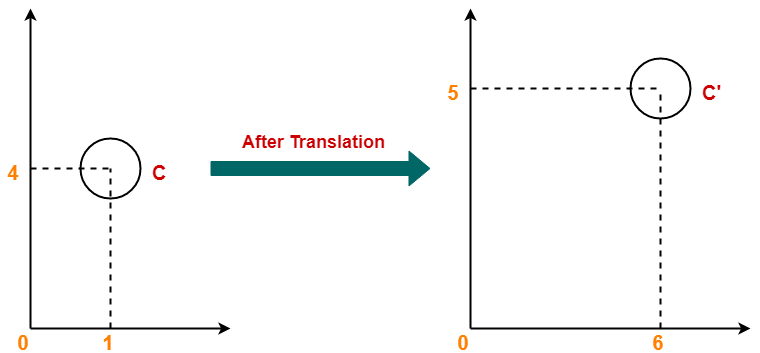## Problem-02:

Given a square with coordinate points A(0, 3), B(3, 3), C(3, 0), D(0, 0). Apply the translation with distance 1 towards X axis and 1 towards Y axis. Obtain the new coordinates of the square.

## Solution-

Given-

• Old coordinates of the square = A (0, 3), B(3, 3), C(3, 0), D(0, 0)
• Translation vector = (Tx, Ty) = (1, 1)

### For Coordinates A(0, 3)

Let the new coordinates of corner A = (Xnew, Ynew).

Applying the translation equations, we have-

• Xnew = Xold + Tx = 0 + 1 = 1
• Ynew = Yold + Ty = 3 + 1 = 4

Thus, New coordinates of corner A = (1, 4).

### For Coordinates B(3, 3)

Let the new coordinates of corner B = (Xnew, Ynew).

Applying the translation equations, we have-

• Xnew = Xold + Tx = 3 + 1 = 4
• Ynew = Yold + Ty = 3 + 1 = 4

Thus, New coordinates of corner B = (4, 4).

### For Coordinates C(3, 0)

Let the new coordinates of corner C = (Xnew, Ynew).

Applying the translation equations, we have-

• Xnew = Xold + Tx = 3 + 1 = 4
• Ynew = Yold + Ty = 0 + 1 = 1

Thus, New coordinates of corner C = (4, 1).

### For Coordinates D(0, 0)

Let the new coordinates of corner D = (Xnew, Ynew).

Applying the translation equations, we have-

• Xnew = Xold + Tx = 0 + 1 = 1
• Ynew = Yold + Ty = 0 + 1 = 1

Thus, New coordinates of corner D = (1, 1).

Thus, New coordinates of the square = A (1, 4), B(4, 4), C(4, 1), D(1, 1).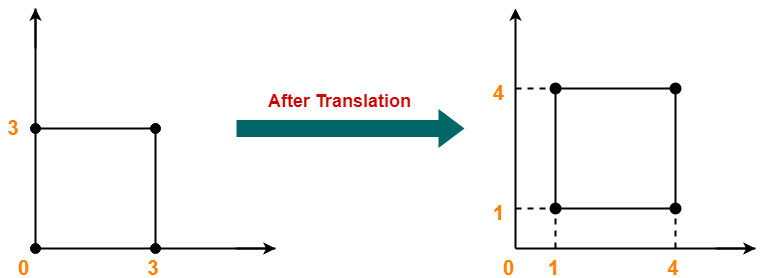To gain better understanding about 2D Translation in Computer Graphics,

Watch this Video Lecture

Next Article- 2D Rotation in Computer Graphics

Get more notes and other study material of Computer Graphics.

Watch video lectures by visiting our YouTube channel LearnVidFun.

SummaryArticle Name
2D Translation in Computer Graphics | Definition | Examples
Description
2D Transformation in Computer Graphics- 2D Translation in Computer Graphics is a process of moving an object from one position to another in 2D plane. Solved Examples and Problems.
Author
Publisher Name
Gate Vidyalay
Publisher Logo